#### Probabilistic Dijkstra

A probabilistic version of Dijkstra's algorithm does not exist in general; however, for some problems, it can be made to work. The algorithm in Figure 10.8 is adapted to the probabilistic case by using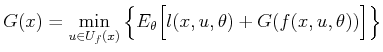(10.64)

in the place of (10.64). The definition of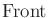remains the same, and the nondeterministic forward projections are still applied to the probabilistic problem. Only edges in the transition graph that have nonzero probability are actually considered as possible future states. Edges with zero probability are precluded from the forward projection because they cannot affect the computed cost values.

The probabilistic version of Dijkstra's algorithm can be successfully applied if there exists a plan,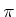, for which from any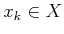there is probability one that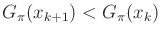. What does this condition mean? From any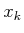, all possible next states that have nonzero probability of occurring must have a lower cost value. If all edge costs are positive, this means that all paths in the multi-stage forward projection will make monotonic progress toward the goal. In the deterministic case, this always occurs if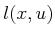is always positive. If nonmonotonic paths are possible, then Dijkstra's algorithm breaks down because the region in which cost-to-go values change is no longer contained within a propagating band, which arises in Dijkstra's algorithm for deterministic problems.

Steven M LaValle 2020-08-14